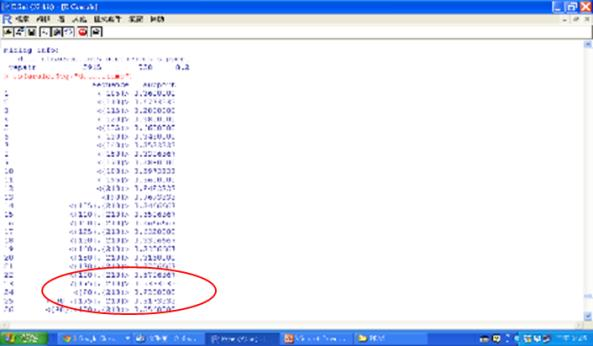ISSN 2077-8813

# R軟體資料探勘實務(中)—關聯模型{Milk, Diaper} a {Beer} (s=0.4, c=0.67)
{Milk, Beer}a{Diaper} (s=0.4, c=1.0)
{Diaper, Beer}a{Milk} (s=0.4, c=0.67)
{Beer}a{Milk, Diaper} (s=0.4, c=0.67)

1. 資料是 0,1 二元檔R軟體的指令如下：
#Apriori beer.xls
library(xlsx)
library(arules)
beer=as.matrix(beer)
rule=apriori(beer,parameter=list(supp=0.2,conf=0.8,maxlen=5))
#default 是0.1, 0.8, 10
inspect(rule)
summary(rule)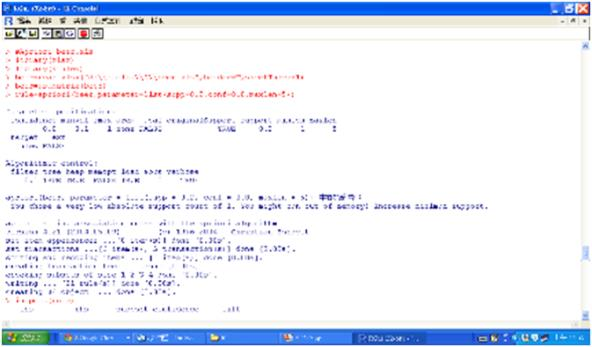2. 若資料格式是各項物品名稱，則輸入後需轉換成交易檔beer2= as(beer, "transactions")library(arules)
beer2=as(beer,"transactions")
beer2
rule=apriori(beer2,parameter=list(supp=0.2,conf=0.8,maxlen=5))R軟體的指令如下：
library(arules)
shopping=shopping[,1:10]
shopping=na.exclude(shopping)
shopping=as.matrix(shopping)
rule=apriori(shopping,parameter=list(supp=0.09, conf=0.8,maxlen=5))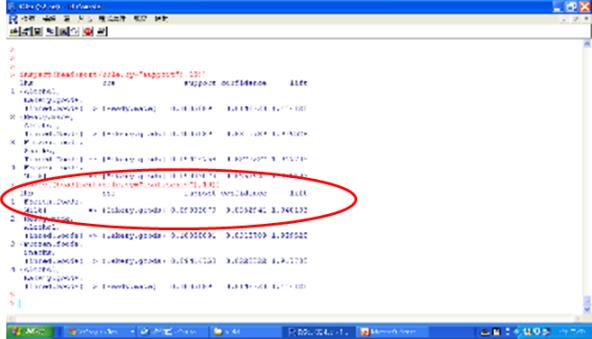df=as.data.frame(Titanic) #32x5
Titan=NULL
for (i in 1:4)
Titan=cbind(Titan,rep(as.character(df[,i]),df\$Freq))
Titan=as.data.frame(Titan) #2201x4
names(Titan)=names(df)[1:4]
summary(Titan)
library(arules)
rule=apriori(Titan)
inspect(rule)rule=apriori(Titan,parameter=list(minlen=2,supp=0.005,conf=0.8),appearance= list(rhs=c("Survived=No","Survived=Yes"),default="lhs"))
rulesort=sort(rule,by="lift")
inspect(rulesort)
subset.matrix=is.subset(rulesort,rulesort)
redundant=colSums(subset.matrix) > 1
which(redundant)
rulepruned=rulesort[!redundant]
inspect(rulepruned)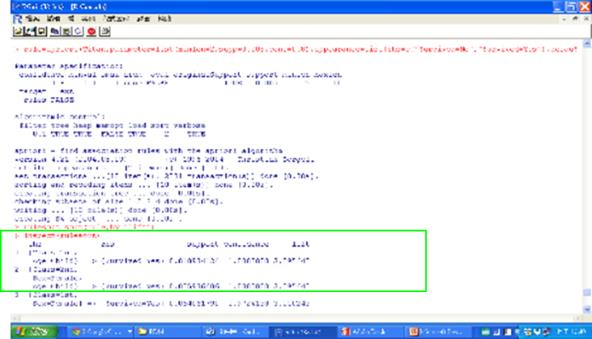rule2=apriori(Titan, control = list(verbose=F),
parameter = list(minlen=3, supp=0.002, conf=0.2),
appearance = list(default="none", rhs=c("Survived=Yes"),
lhs=c("Class=1st", "Class=2nd", "Class=3rd", "Age=Child", "Age=Adult")))
rule2.sorted= sort(rule2, by="confidence")
inspect(rule2.sorted)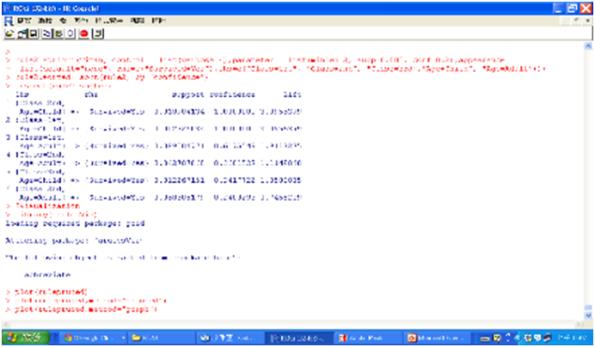library(arulesViz)
#Heat map (熱圖)
plot(rulepruned)
#Balloon plot (氣球圖)
plot(rulepruned,method="grouped")
# Graph (網路圖)
plot(rulepruned,method="graph",control =list(type="items"))
# Parallel coordinates plot (平行座標圖)
plot(rulepruned, method = "paracoord", control = list(reorder = TRUE))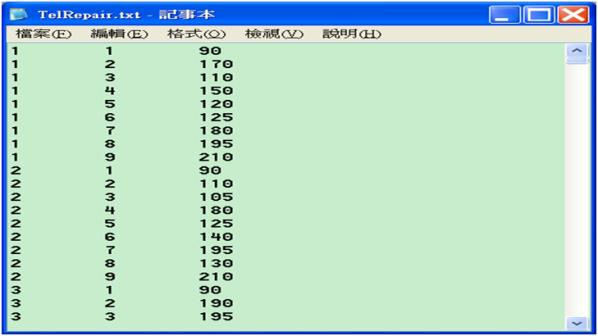library("arulesSequences")